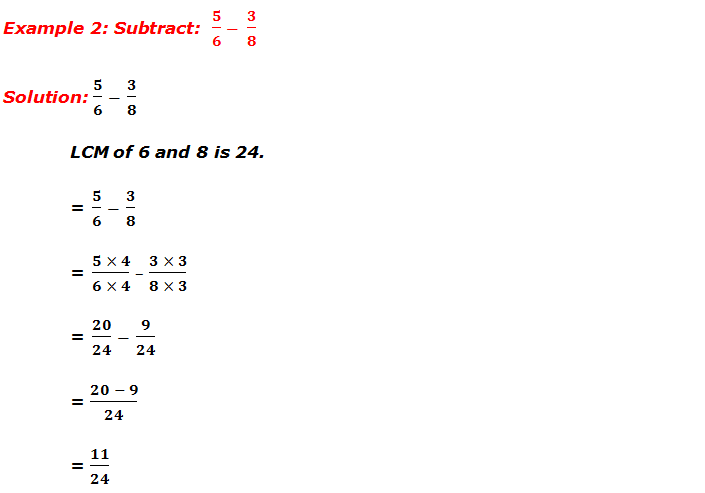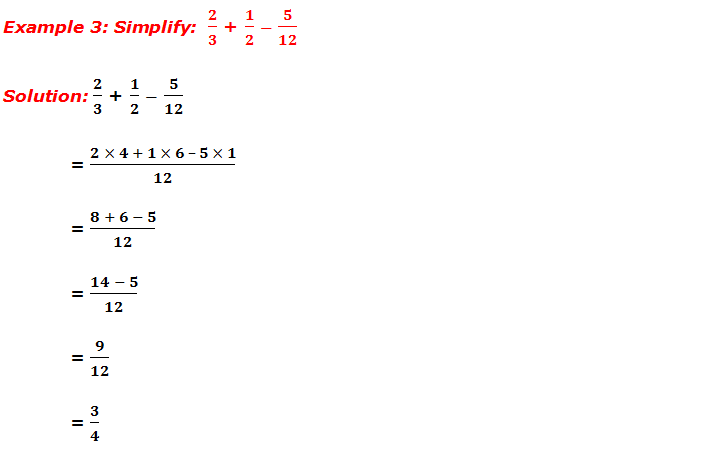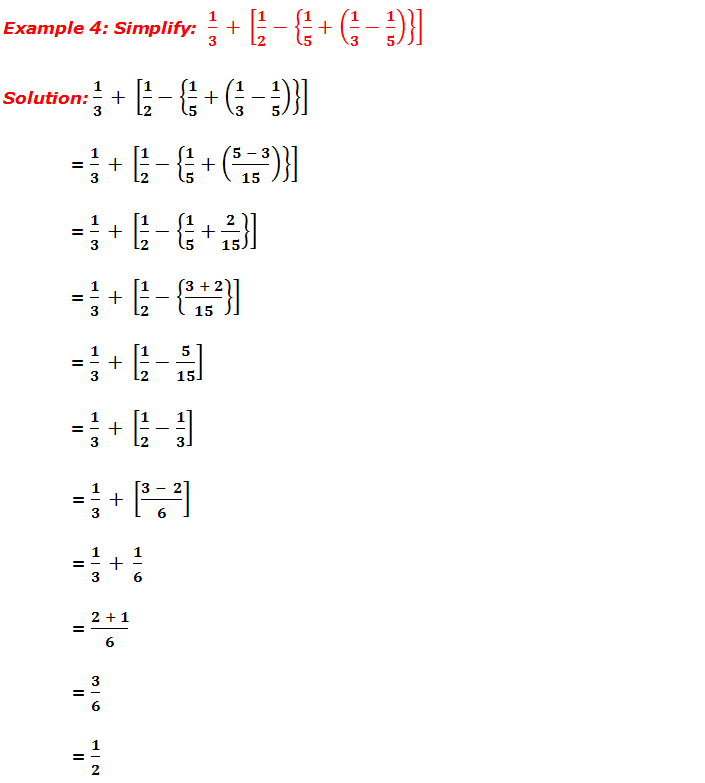# Addition, Subtraction and Simplification of Fractions

### Addition and subtraction of like fraction

When adding or subtracting like fractions, we add or subtract the numerators of the like fractions and keep the denominator as it is.

********************

10 Math Problems officially announces the release of Quick Math Solver, an Android App on the Google Play Store for students around the world.

********************

For example:

### Addition and subtraction of unlike fractions

When adding or subtracting unlike fractions we take the LCM of the denominators and convert the fractions into like fractions, then simplify.For example, the denominators of the fractions given below are 4 and 3. The LCM of 4 and 3 is 12. Then the given fractions are converted into like fractions and simplified as given below.

## Simplification of Fractions

While simplifying fractions under the relation of basic operations ÷, ×, +, - and with brackets, we need to follow the BODMAS rule.

 1 B Brackets [{()}] 2 O Of × 3 D Division ÷ 4 M Multiplication × 5 A Addition + 6 S Subtraction -

The following worked out examples will give the concepts of addition, subtraction and simplification of the fractions.

## Workout ExamplesYou can comment your questions or problems regarding the addition, subtraction and simplification of fractions here.